# Using the COUNT Functions

In this article, we will learn about the Count family function in Microsoft Excel. How and where we use it in data?

We have 5 types of count functions in Microsoft Excel.

• COUNT
• COUNTA
• COUNTBLANK
• COUNTIF
• COUNTIFS
Formula Syntax Condition
COUNT =COUNT(value1, [value2],[value3],…) Count the cells which contain value
COUNTA =COUNTA(value1, [value2],[value3],…) Count the non-empty cells
COUNTBLANK =COUNTBLANK(range) Count the empty cells
COUNTIF =COUNTIF(range, criteria) Count the condition based value for single criteria
COUNTIFS =COUNTIFS (criteria_range1, criteria1, [criteria_range2, criteria2],…) Count the condition based value for multiple criteria’s

COUNT Function:-

Let’s take an example and understand

We have credit card's billing data and, in the credit card column, we want to count the cells that contain some value.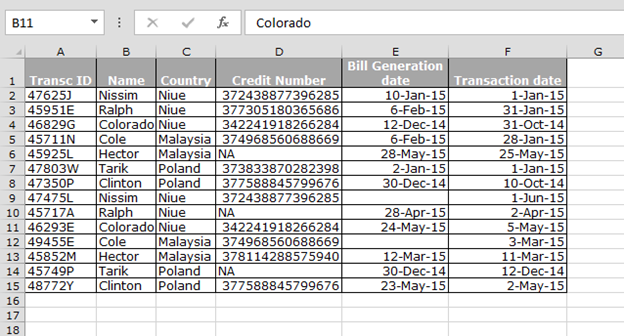• Enter the formula in cell G2.
• =COUNT(D2:D15)
• Press Enter.
• The function will return 11.
• Total cells were 14, and we got the result 11. It means 11 cells contain integer value out of 14 cells.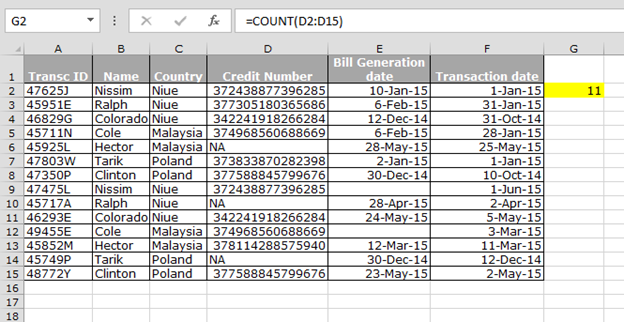Conclusion: - We use COUNT function when we want to count only numbers containing cells.

COUNTA Function:-

Let’s take an example and understand

We have credit card’s data and, in the bill generation date range, we want to count non-empty cells.• Enter the formula in cell G2.
• =COUNTA(E2:E15)
• Press Enter.
• The function will return 12.
• Total cells were 14, and we got the result 12. It means 12 cells are non-empty out of 14 cells.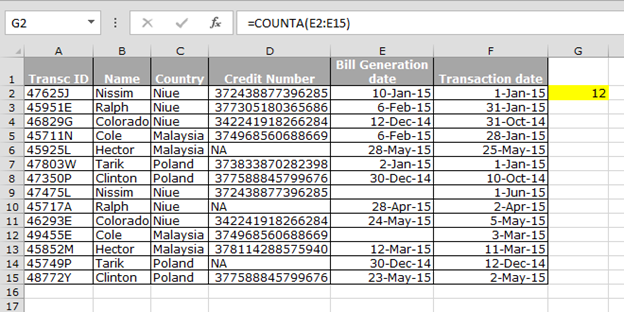Conclusion: - We use COUNTA function to count the non-empty cells in the range.

COUNTBLANK Function:-

Let’s take an example and understand

We have credit card’s data and,in the bill generation date range, we want to count empty cells.• Enter the formula in cell G2.
• =COUNTBLANK(E2:E15)
• Press Enter.
• The function will return 2.
• Total cells were 14, and we got the result 2. It means 2 cells are empty out of 14 cells.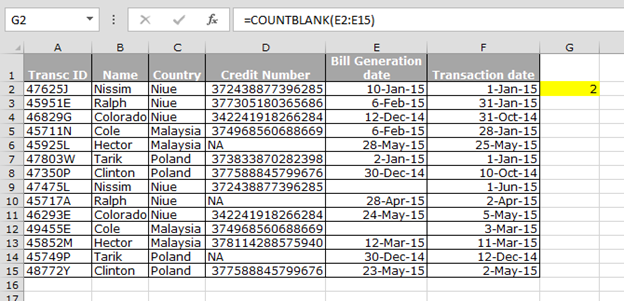Conclusion: - We use COUNTBLANK function to count the empty cells in the range.

COUNTIF Function:-

Let’s take an example and understand

We have credit card’s data and from that data, we want to count the number of bills of the country, Niue.• Enter the formula in cell G2.
• =COUNTIF(\$C\$2:\$C\$15,"Niue")
• Press Enter.
• The function will return 6.
• Niue’s three bills are in the data.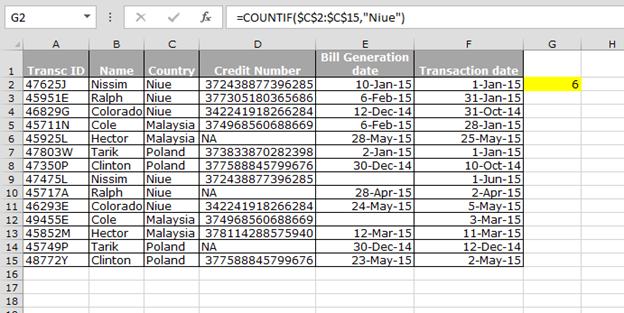Conclusion: - We use COUNTIF function to count the data based on single criteria or we can say that we can count the number of times the value repeats in the range.

COUNTIFS Function:-

Let’s take an example and understand

We have credit card’s data and, from that data, we want to count the number of bills of Nissim.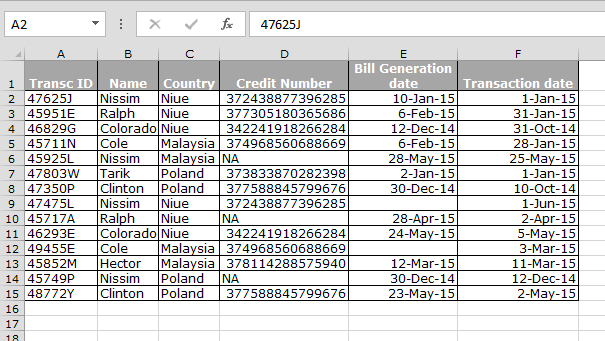• Enter the formula in cell G2.
• =COUNTIFS(C2:C15,"Niue",B2:B15,"Nissim")
• Press Enter.
• The function will return 2.
• It means after Niue’s country, Nissim is repeating 2 times.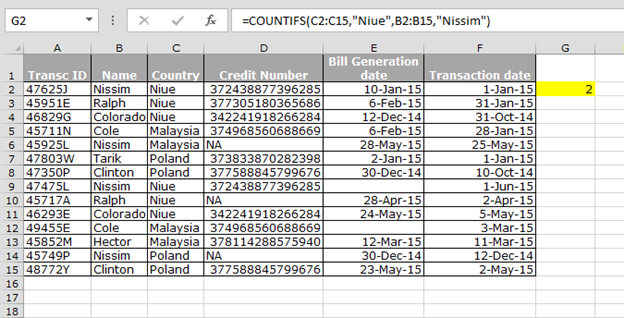Conclusion: - We use COUNTIFS function to count the data based on multiple criteria in the data.

These are the COUNT functions in Microsoft Excel which we use to solve different types of problems.